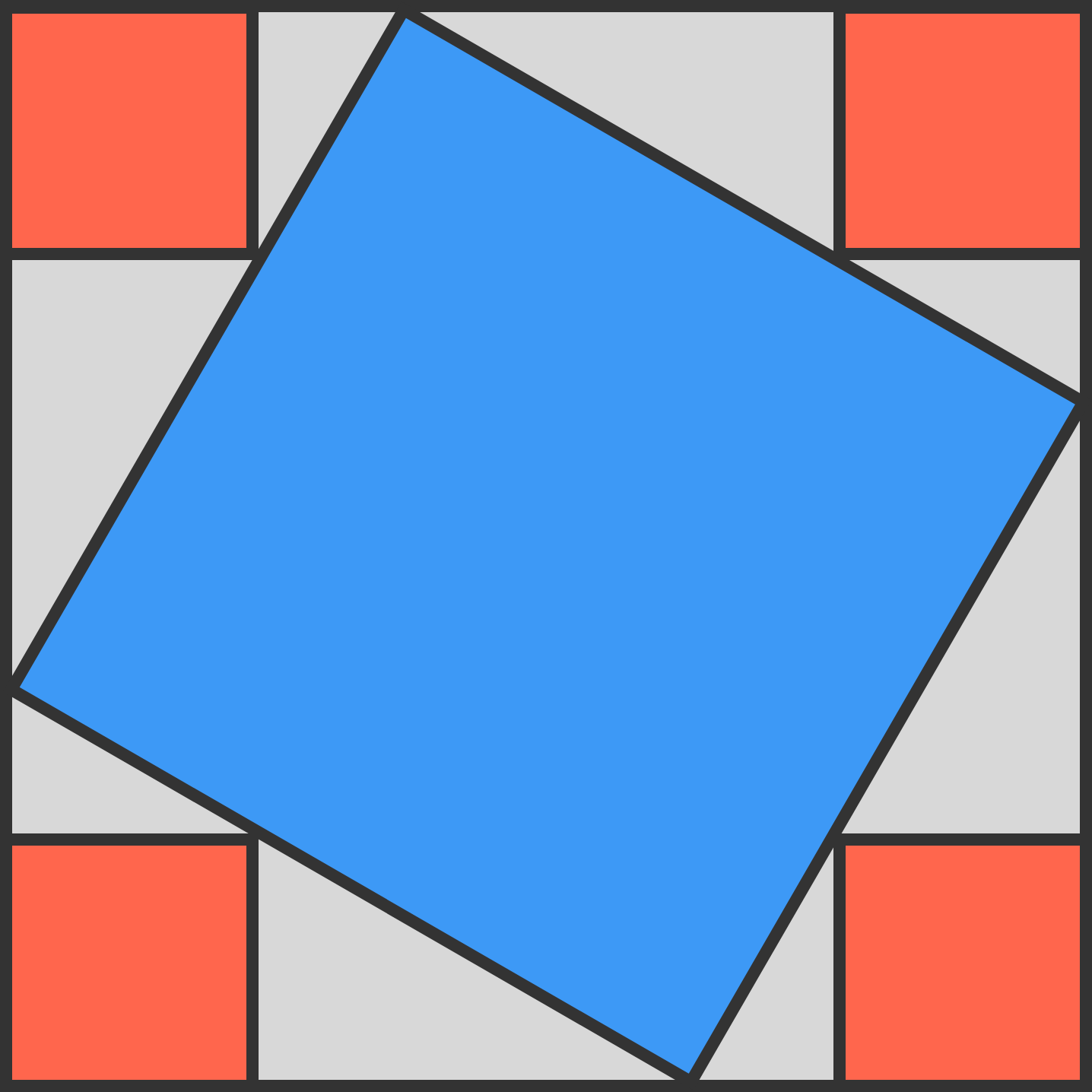# Square ratiosThe blue square touches each side of the largest square, filling $\frac{29}{50}$ of it.

Each of the 4 red squares at the corners of the largest square touch the blue square.

The fraction of the largest square that is red is $\frac{a}{b},$ where $a$ and $b$ are positive coprime integers.

What is $a+b?$

×